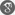##### Cite as:
E.U. Ofoedu, Agatha Chizoba Nnubia, Convergence Theorem for Finite Family of Total Asymptotically Nonexpansive Mappings, Int. J. Anal. Appl., 9 (2) (2015), 96-113.

#### Abstract

In this paper we introduce an explicit iteration process and prove strong convergence of the scheme in a real Hilbert space $H$ to the common fixed point of finite family of total asymptotically nonexpansive mappings which is nearest to the point $u \in H$.  Our results improve previously known ones obtained for the class of asymptotically nonexpansive mappings. As application, iterative method for: approximation of solution of variational Inequality problem, finite family of continuous pseudocontractive mappings, approximation of solutions of classical equilibrium problems and approximation of solutions of convex minimization problems are proposed. Our theorems unify and complement many recently announced results.

#### References

1. Y. Alber, R. Espinola and P. Lorenzo, Strongly Convergent Approximations to fixed points of total asymptotically nonexpansive mappings, Acta Mathematica Sinica, English Series, vol. 24 no. 6 (2008) 1005-1022.2. Ya. Alber, C. E. Chidume and H. Zegeye, Approximating fixed points of total asymptotically nonexpansive mappings.Fixed Point Theory and Appl. 2006 (2006), article ID 10673.3. H. H. Bauschke, The Approximation of fixed points of compositions of nonexpansive mappings in Hilbert spaces, J. Math. Anal. Appl. 202 (1996) 150-159.4. E. Blum and W. Oettli, From optimization and variational inequalities to equilibrum problems, The Mathematics Student 63 Nos. 1-4 (1994), 123-145.5. F. E. Browder, Convergence theorems for sequences of nonlinear operators in Banach spaces, Math. Zeitschr. 100 (1967) 201-225.6. C. Byrne, Iterative oblique projection onto convex subsets and split feasibility problems, inverse problems, 18(2002)441-453.7. Y. Censor, T. Bortfeld. B.Martin and Trofimov, A unified approach for inversion problem in intensity modulated radiation therapy,Pys.Med. Biol., 51(2006) 2353-2365.8. Y. Censor and Elfving, A multiprojection algorithm using Bregman projection in a product space, Numer. Algorithms, 8(1994) 221-239.9. C.E. Chidume; Geometric properties of Banach spaces and nonlinear iterations, Springer Verlag Series: Lecture Notes in Mathematics Vol. 1965 (2009).10. C. E. Chidume, Jinlu Li and A. Udomene; Convergence of paths and approximation of fixed points of asymptotically nonexpansive mappings, Proc. Amer. Math. Soc., 133 (2005), 473- 480.11. K. Goebel and Kirk, A fixed point theorem for asymptotically nonexpansive mappings Proc. Amer. Math. Soc. 35(1972),171-174.12. B. Halpern, Fixed point of nonexpansive maps, Bull.Amer. Math. Soc., 73(1967), 975-961.13. S. Ishikawa; Fixed point by a new iteration method, Proc. Amer. Math. Soc. 44(1974) 147-150.14. P. Katchang, T. Jitpeera and P. Kumam, Strong convergence theorems for solving generalized mixed equilibrium problems and general system of variational inequalities by the hybrid method, Nonlinear Analysis: hybrid systems 4 (2010) 838C852.15. T.C.Lim and H.K. Xu Fixed point theorems for asymtotically nonexpansive mappings, Nonlinear Anal., 22(1994), 1345-1355.16. P. L. Lions, Approximation de points fixes de contractions, Computes rendus de l’academie des sciences, serie I-mathematique, 284 (1997) 1357-1359.17. P.E Mainge, Strong Convergence of projected subgradient method for non smooth and non strickly convex minimizaton, Set Valued Anal. 16 (2008)899-912.18. E. U. Ofoedu, A General Approximation Scheme for Solutions of Various Problems in Fixed Point Theory, International Journal of Analysis, 2013 (2013), Article ID 762831.19. E. U. Ofoedu and H. Zegeye, Further investigation on iteration processes for pseudocontractive mappings with application, Nonlinear Anal. TMA 75 (2012) 153-162.20. Ofoedu, E. U. and Madu, L. O. Iterative Procedure For Finite Family Of Total asymptotically nonexpansive mappings,Journal of the Nigerian Mathematical Society, 33(2014) 93-112.21. E.U. Ofoedu and Agatha C. Nnubia, Strong Convergence Theorem for Minimum-norm Fixed Point of Total asymptotically Nonexpansive Mapping. Afrika Matematika(2014) Doi: 1007/513370-014-0240-4.22. J.G Ohara p. pillary and H.K. XU, iterative appraoches to convex feasibility problem in benach spaces, Nonlinear anal., 64(20060, 2022-2042.23. M.O Osilike, E. E.Chima. P.U. Nwokoro and F. U. Ogbuisi, Strong Convergence of a Modified Averaging Iterative algorithm for asymptotically nonexpansive maps, Journal of the Nigerian Mathematical Society, 32(2013), 241-251.24. S. Reich. S.Reich, Strong Convergence theorems for resolvents of accretive operators in benach spaces, J.Math. Anal. Appl. (1966), 276-284.25. W Takahashi, Nonlinear Functional Analysis- Fixed point theory and applications, Yokohanna publisher inc. Yokohanna (2000).26. R. Wittmann Approximation of fixed point of nonexpansive mappings ,Arc Math,58(1999) 486-49127. X. Yang, Y. C Liou and Y. Yao Finding minimum norm fixed point of nonexpansive mappings and applications, Mathematical problems in engineering, V. 2011, Article ID 106450.28. Y. Yao, H.K Xu and Y.C Liou, Strong Convergence of a Modified KrasnoselskiMann Iterative algorithm for non-expansive mapping, J. Appl. Comput. 29(2009) 383-389.29. H. Zegeye, An iterativee approximation method for a common fixed point of two pseudocontractive mappings, ISRN Math. Anal. 14 (2011). Article ID 621901.30. H. Zegeye, E. U. Ofoedu and N. Shahzad, Convergence theorems for equilibrum problem,variational inequality problem and countably infinite relatively quasi-nonexpansive mappings,Applied Mathematics and Computation, 216 (2010) 3439-344931. H. Zegeye and N. Shahzad, Approximation of the common minimum-norm fixed point of a finite family of asymptotically nonexpansive mappings, Fixed Point Theory and Appl. 2013 (2013) Article ID 1.